Phases of Stellar Evolution Phases of Stellar Evolution

• Slides: 60Phases of Stellar Evolution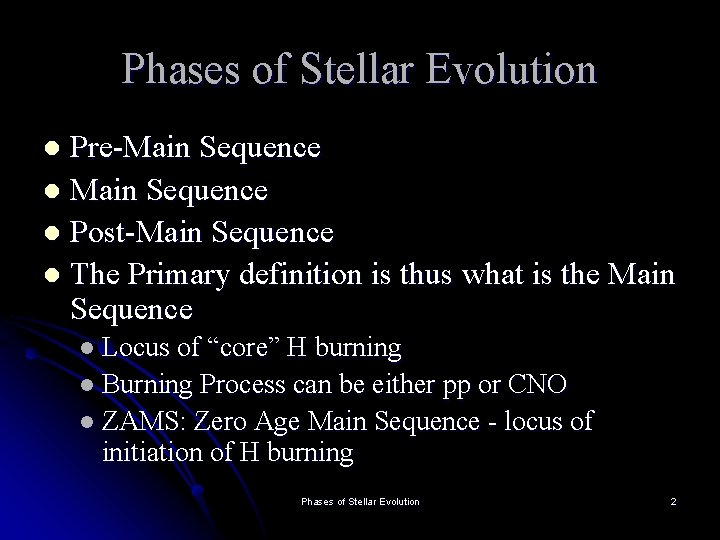Phases of Stellar Evolution Pre-Main Sequence l Post-Main Sequence l The Primary definition is thus what is the Main Sequence l l Locus of “core” H burning l Burning Process can be either pp or CNO l ZAMS: Zero Age Main Sequence - locus of initiation of H burning Phases of Stellar Evolution 2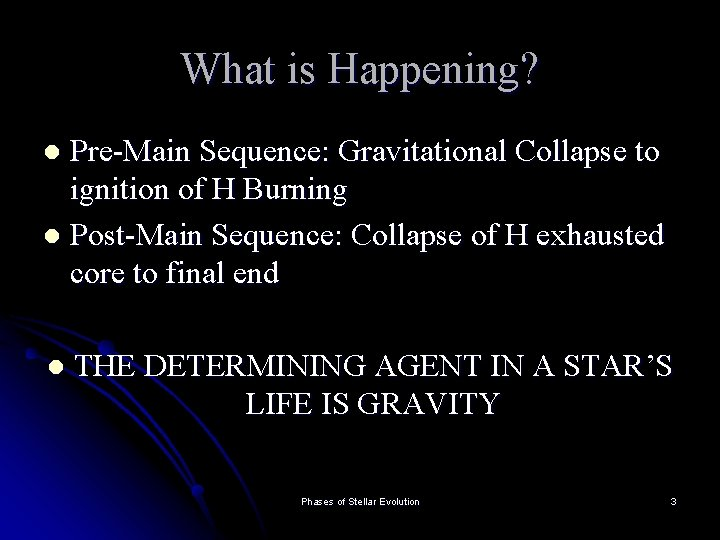What is Happening? Pre-Main Sequence: Gravitational Collapse to ignition of H Burning l Post-Main Sequence: Collapse of H exhausted core to final end l l THE DETERMINING AGENT IN A STAR’S LIFE IS GRAVITY Phases of Stellar Evolution 3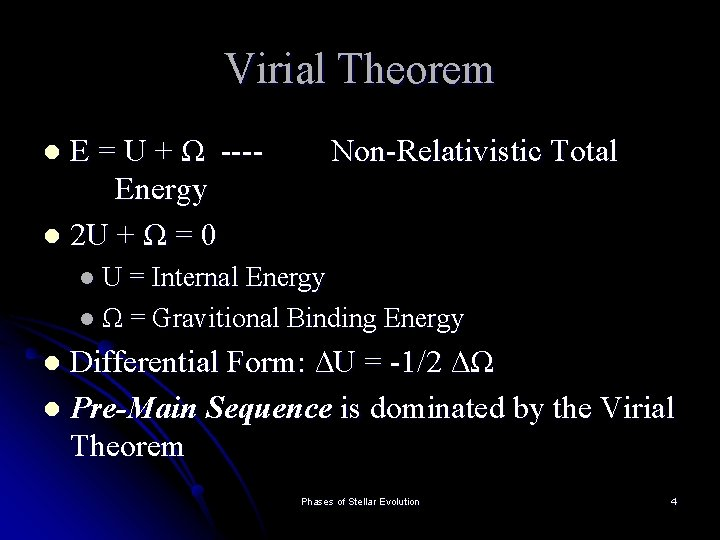Virial Theorem E = U + Ω ---Energy l 2 U + Ω = 0 Non-Relativistic Total l l U = Internal Energy l Ω = Gravitional Binding Energy Differential Form: ∆U = -1/2 ∆Ω l Pre-Main Sequence is dominated by the Virial Theorem l Phases of Stellar Evolution 4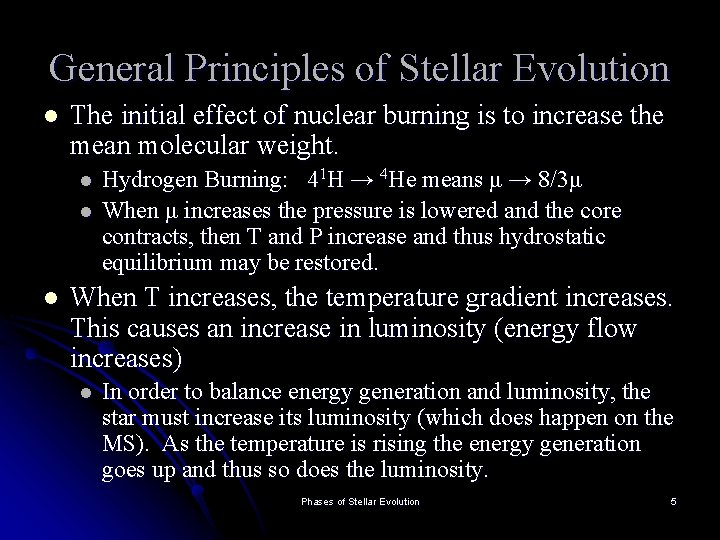General Principles of Stellar Evolution l The initial effect of nuclear burning is to increase the mean molecular weight. l l l Hydrogen Burning: 41 H → 4 He means μ → 8/3μ When μ increases the pressure is lowered and the core contracts, then T and P increase and thus hydrostatic equilibrium may be restored. When T increases, the temperature gradient increases. This causes an increase in luminosity (energy flow increases) l In order to balance energy generation and luminosity, the star must increase its luminosity (which does happen on the MS). As the temperature is rising the energy generation goes up and thus so does the luminosity. Phases of Stellar Evolution 5Main Sequence Configuration Thermonuclear core (pp/CNO) The core is either convective (CNO burners) or radiative (pp burners) Envelope: Convective or Radiative (Opposite of core). Phases of Stellar Evolution 6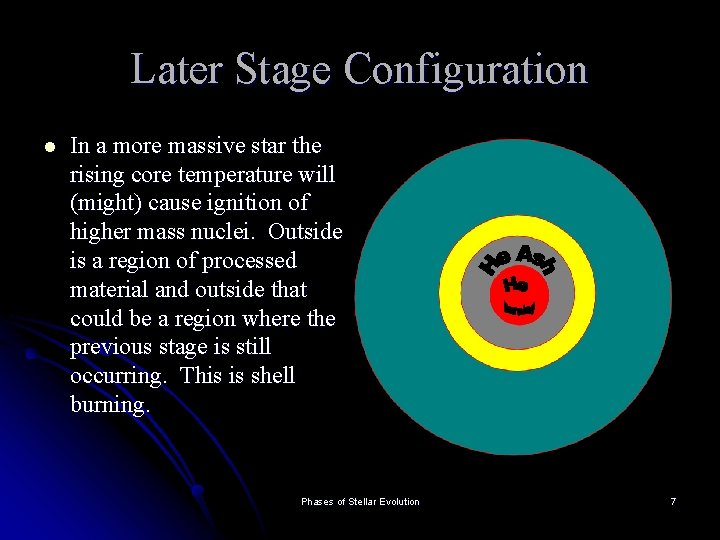Later Stage Configuration l In a more massive star the rising core temperature will (might) cause ignition of higher mass nuclei. Outside is a region of processed material and outside that could be a region where the previous stage is still occurring. This is shell burning. Phases of Stellar Evolution 7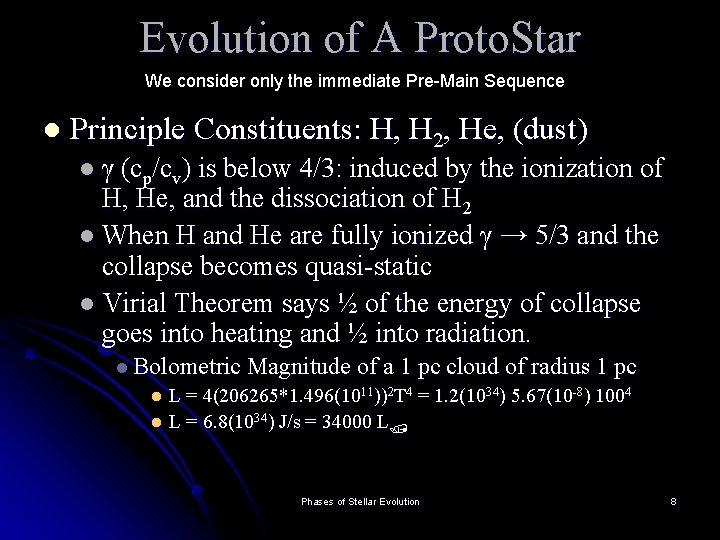Evolution of A Proto. Star We consider only the immediate Pre-Main Sequence l Principle Constituents: H, H 2, He, (dust) l γ (cp/cv) is below 4/3: induced by the ionization of H, He, and the dissociation of H 2 l When H and He are fully ionized γ → 5/3 and the collapse becomes quasi-static l Virial Theorem says ½ of the energy of collapse goes into heating and ½ into radiation. l Bolometric Magnitude of a 1 pc cloud of radius 1 pc L = 4(206265*1. 496(1011))2 T 4 = 1. 2(1034) 5. 67(10 -8) 1004 l L = 6. 8(1034) J/s = 34000 L l Phases of Stellar Evolution 8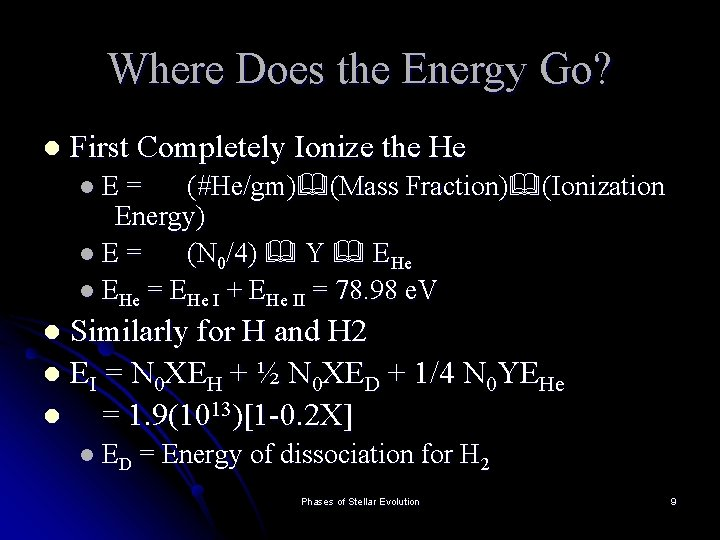Where Does the Energy Go? l First Completely Ionize the He (#He/gm) (Mass Fraction) (Ionization Energy) l. E= (N 0/4) Y EHe l EHe = EHe I + EHe II = 78. 98 e. V l. E= Similarly for H and H 2 l EI = N 0 XEH + ½ N 0 XED + 1/4 N 0 YEHe l = 1. 9(1013)[1 -0. 2 X] l l ED = Energy of dissociation for H 2 Phases of Stellar Evolution 9Internal Energy l l From the Virial Theorem MEI ½ α GM 2/R (M is the Mass collapsing) l l α depends on the order of the polytrope n = 1. 5 (γ = 5/3) α = 6/7 α is always of order unity Now solve for the radius: l l R/R = 43. 2(M/M ) / [1 - 0. 2 X] This is the maximum radius for a stable star at the beginning of its evolution If larger then ionization and disassociation will not be complete. Once 43. 2(M/M ) / [1 - 0. 2 X] is achieved quasistatic evolution is possible. Phases of Stellar Evolution 10What is the Central Temperature? l l Tc = 3(105)μ(1 -0. 2 X) This is always less than ignition temperature (107 K) so the energy source is gravitational collapse. ET = Total Energy of the Star Luminosity = Energy Flow / Time = d. ET / dt Phases of Stellar Evolution 11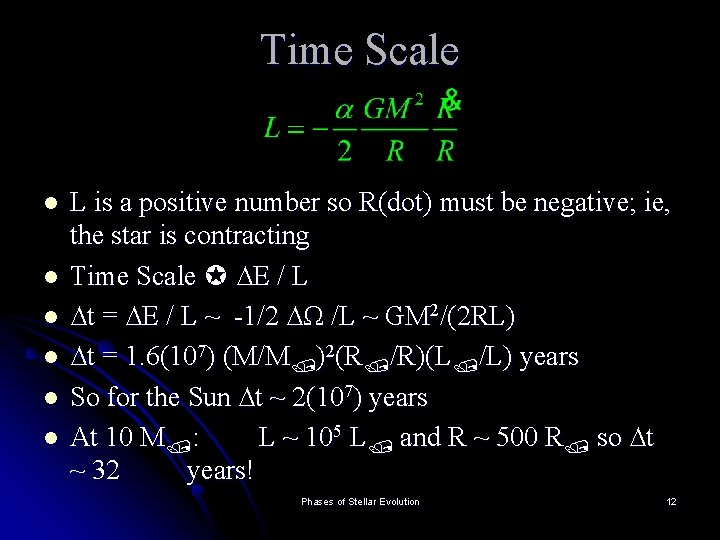Time Scale l l l L is a positive number so R(dot) must be negative; ie, the star is contracting Time Scale ∆E / L ∆t = ∆E / L ~ -1/2 ∆Ω /L ~ GM 2/(2 RL) ∆t = 1. 6(107) (M/M )2(R /R)(L /L) years So for the Sun ∆t ~ 2(107) years At 10 M : L ~ 105 L and R ~ 500 R so ∆t ~ 32 years! Phases of Stellar Evolution 12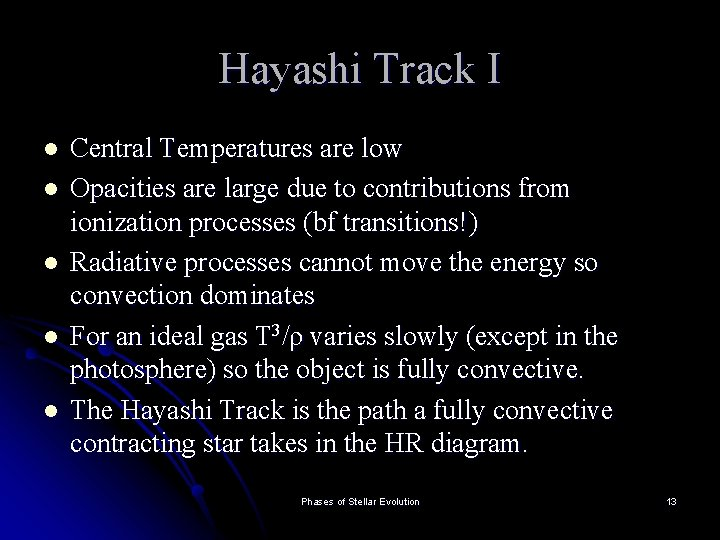Hayashi Track I l l l Central Temperatures are low Opacities are large due to contributions from ionization processes (bf transitions!) Radiative processes cannot move the energy so convection dominates For an ideal gas T 3/ρ varies slowly (except in the photosphere) so the object is fully convective. The Hayashi Track is the path a fully convective contracting star takes in the HR diagram. Phases of Stellar Evolution 13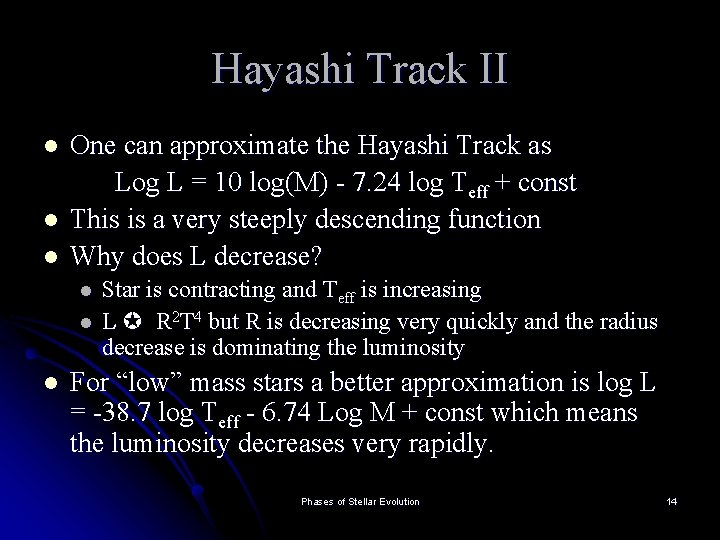Hayashi Track II l l l One can approximate the Hayashi Track as Log L = 10 log(M) - 7. 24 log Teff + const This is a very steeply descending function Why does L decrease? l l l Star is contracting and Teff is increasing L R 2 T 4 but R is decreasing very quickly and the radius decrease is dominating the luminosity For “low” mass stars a better approximation is log L = -38. 7 log Teff - 6. 74 Log M + const which means the luminosity decreases very rapidly. Phases of Stellar Evolution 14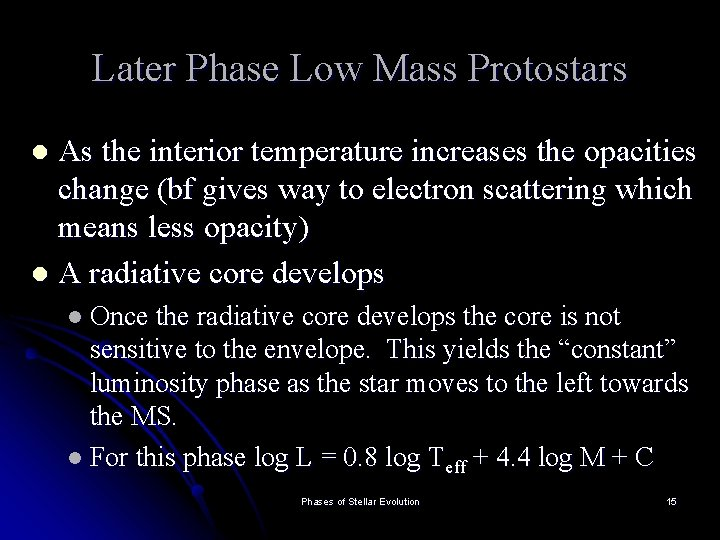Later Phase Low Mass Protostars As the interior temperature increases the opacities change (bf gives way to electron scattering which means less opacity) l A radiative core develops l l Once the radiative core develops the core is not sensitive to the envelope. This yields the “constant” luminosity phase as the star moves to the left towards the MS. l For this phase log L = 0. 8 log Teff + 4. 4 log M + C Phases of Stellar Evolution 15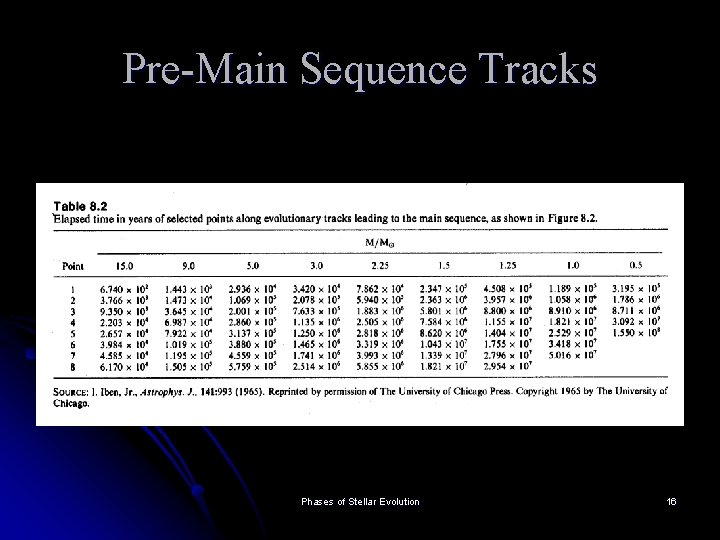Pre-Main Sequence Tracks Phases of Stellar Evolution 16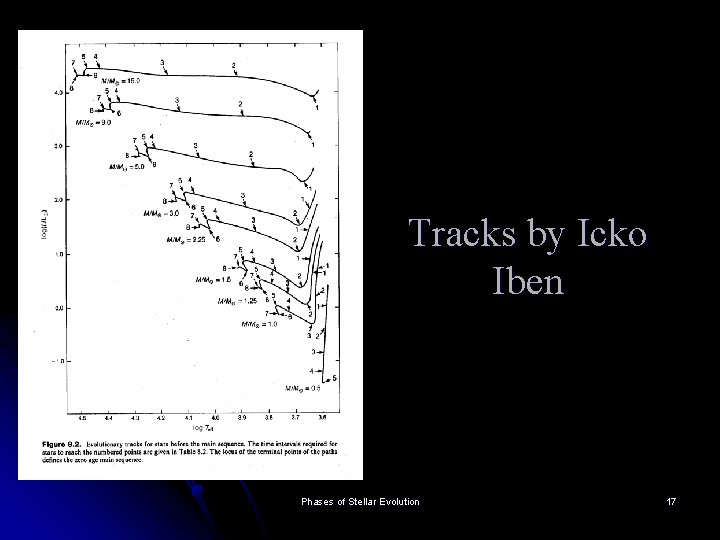Tracks by Icko Iben Phases of Stellar Evolution 17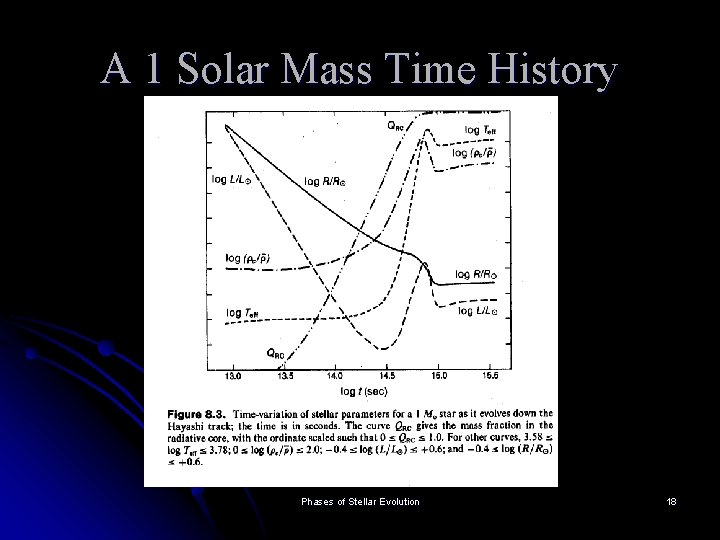A 1 Solar Mass Time History Phases of Stellar Evolution 18NGC 2264 Phases of Stellar Evolution 19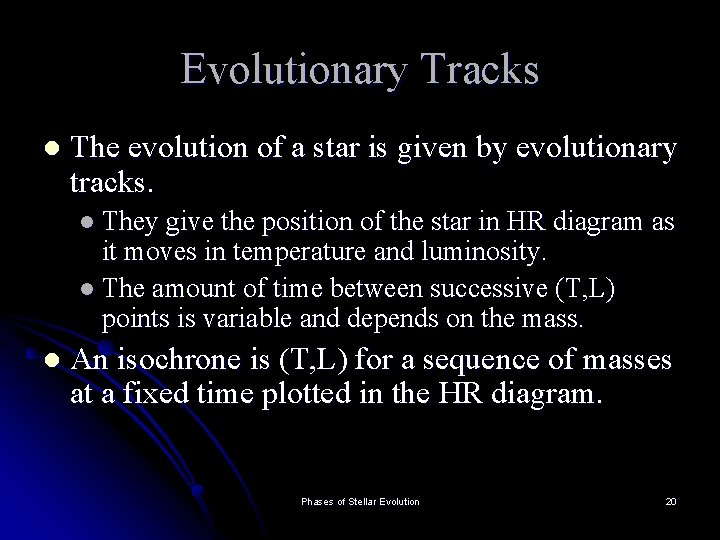Evolutionary Tracks l The evolution of a star is given by evolutionary tracks. l They give the position of the star in HR diagram as it moves in temperature and luminosity. l The amount of time between successive (T, L) points is variable and depends on the mass. l An isochrone is (T, L) for a sequence of masses at a fixed time plotted in the HR diagram. Phases of Stellar Evolution 20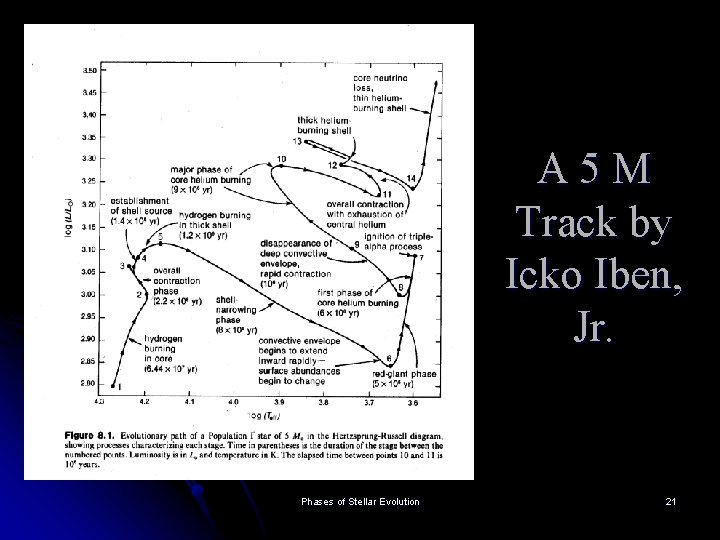A 5 M Track by Icko Iben, Jr. Phases of Stellar Evolution 21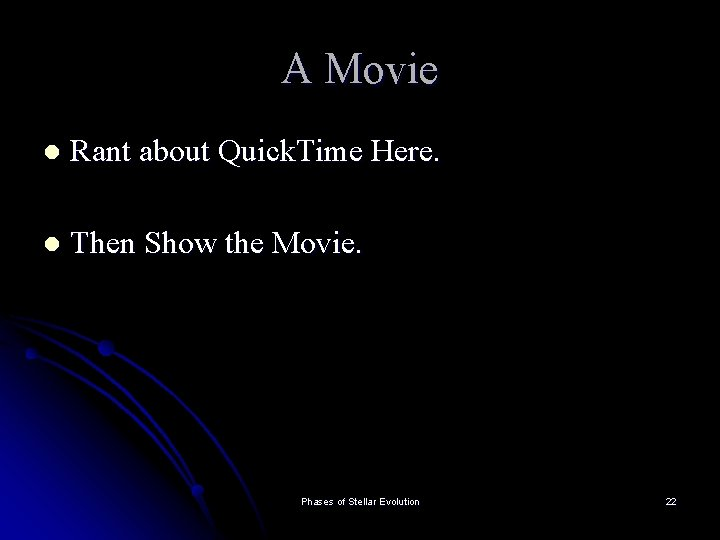A Movie l Rant about Quick. Time Here. l Then Show the Movie. Phases of Stellar Evolution 22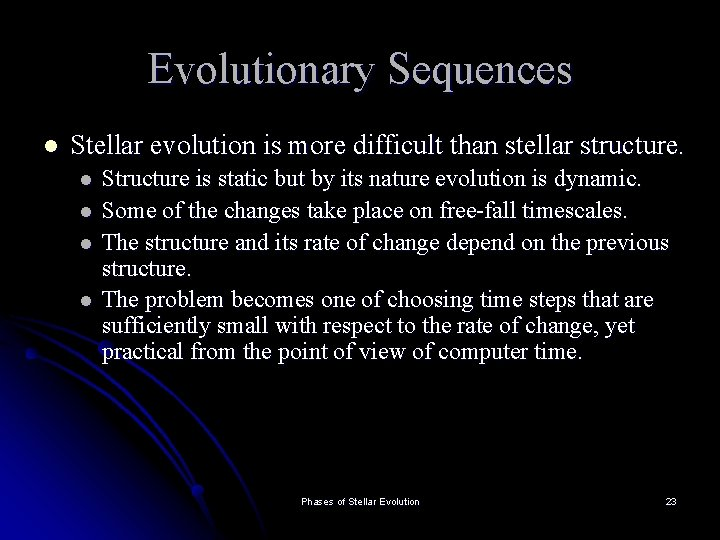Evolutionary Sequences l Stellar evolution is more difficult than stellar structure. l l Structure is static but by its nature evolution is dynamic. Some of the changes take place on free-fall timescales. The structure and its rate of change depend on the previous structure. The problem becomes one of choosing time steps that are sufficiently small with respect to the rate of change, yet practical from the point of view of computer time. Phases of Stellar Evolution 23Stellar Structure Equations Time Dependent Versions l Hydrodynamic Equilibrium l Mass Continuity l Energy Flow l Energy Generation Phases of Stellar Evolution 24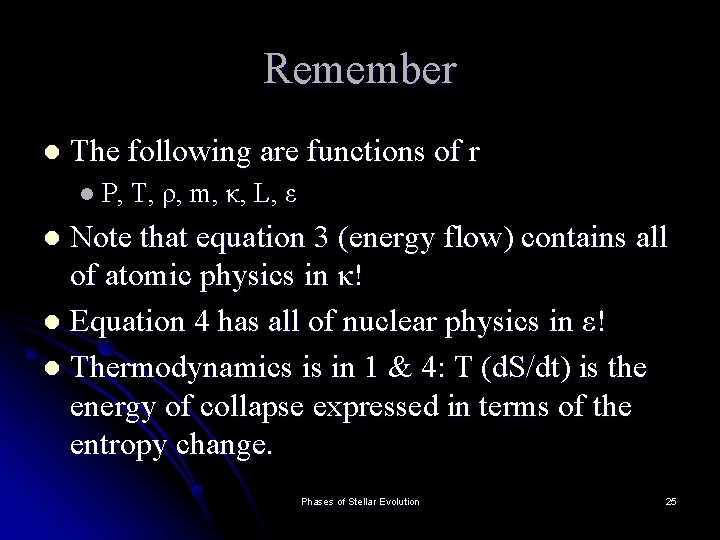Remember l The following are functions of r l P, T, ρ, m, κ, L, ε Note that equation 3 (energy flow) contains all of atomic physics in κ! l Equation 4 has all of nuclear physics in ε! l Thermodynamics is in 1 & 4: T (d. S/dt) is the energy of collapse expressed in terms of the entropy change. l Phases of Stellar Evolution 25The Main Sequence ZAMS: Zero Age Main Sequence l l l Note that the observational MS has a finite width due to the admixture of ages. As a star evolves on the MS it evolves up in luminosity and down in T The dividing point for the energy generation cycles occurs at about 2(107) K l l < 2(107) K uses pp with radiative core and convective envelope > 2(107) K uses CNO with convective core and radiative envelope. Phases of Stellar Evolution 26Consider the CNO Cycle l l For 1. 2(107) K < T < 5(107) K the energy generation goes as 20 n 13 in ε = ε 0ρTn This means that the star will develop a very large temperature gradient due to the sensitivity of the energy generation to T l l l To see this: ∂L/∂r ε and ∂T/∂r L This also means these stars have a highly centralized core in terms of energy generation: a 2% decrease in T yields a 33% decrease in energy generation (n=20) Large temperature gradients means convection which dominates CNO cores Phases of Stellar Evolution 27For the pp Cycle l For the pp cycle at 4(106) < T < 2. 4(107) K l 6 n 3. 5 in ε= ε 0ρTn l This means that the temperature gradient is much smaller. There is less centralization in the energy generation and little tendency for convection in the core. l pp cores are radiative Phases of Stellar Evolution 28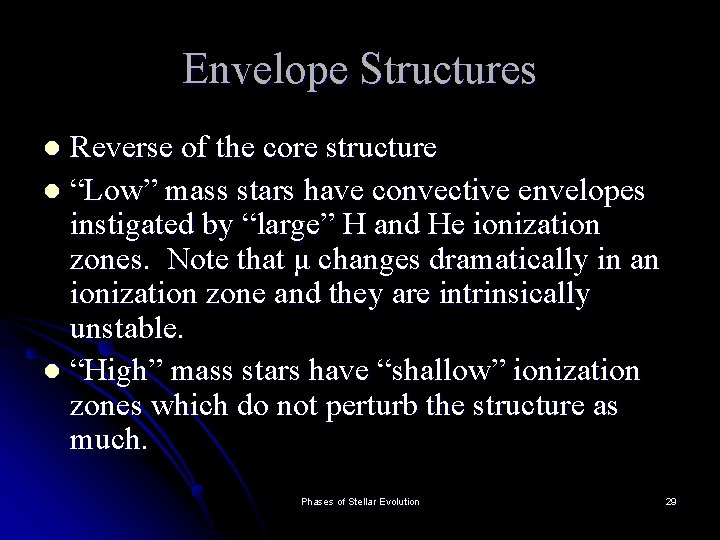Envelope Structures Reverse of the core structure l “Low” mass stars have convective envelopes instigated by “large” H and He ionization zones. Note that μ changes dramatically in an ionization zone and they are intrinsically unstable. l “High” mass stars have “shallow” ionization zones which do not perturb the structure as much. l Phases of Stellar Evolution 29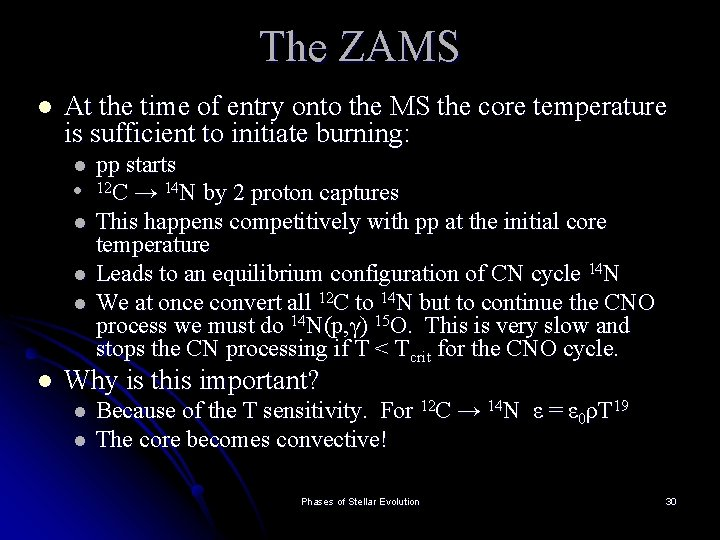The ZAMS l At the time of entry onto the MS the core temperature is sufficient to initiate burning: l l l pp starts 12 C → 14 N by 2 proton captures This happens competitively with pp at the initial core temperature Leads to an equilibrium configuration of CN cycle 14 N We at once convert all 12 C to 14 N but to continue the CNO process we must do 14 N(p, γ) 15 O. This is very slow and stops the CN processing if T < Tcrit for the CNO cycle. Why is this important? l l Because of the T sensitivity. For 12 C → 14 N ε = ε 0ρT 19 The core becomes convective! Phases of Stellar Evolution 30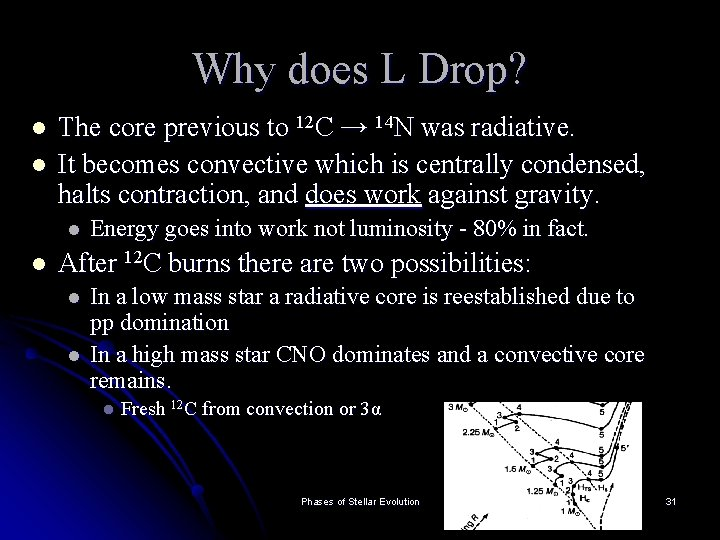Why does L Drop? l l The core previous to 12 C → 14 N was radiative. It becomes convective which is centrally condensed, halts contraction, and does work against gravity. l l Energy goes into work not luminosity - 80% in fact. After 12 C burns there are two possibilities: l l In a low mass star a radiative core is reestablished due to pp domination In a high mass star CNO dominates and a convective core remains. l Fresh 12 C from convection or 3α Phases of Stellar Evolution 31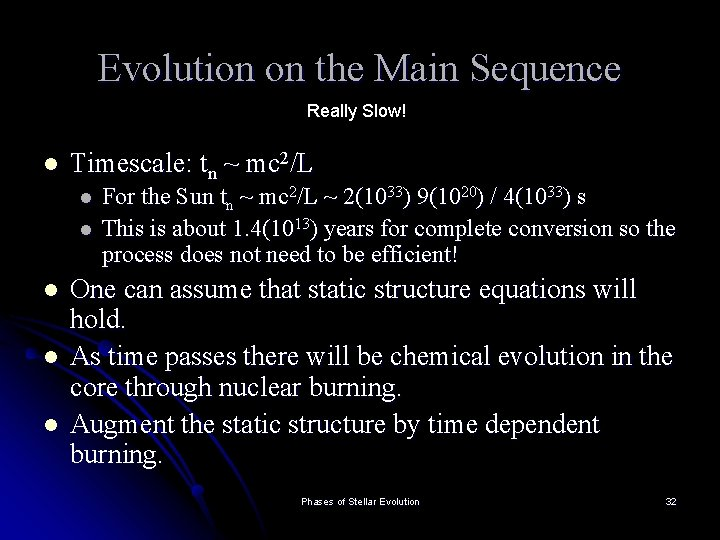Evolution on the Main Sequence Really Slow! l Timescale: tn ~ mc 2/L l l l For the Sun tn ~ mc 2/L ~ 2(1033) 9(1020) / 4(1033) s This is about 1. 4(1013) years for complete conversion so the process does not need to be efficient! One can assume that static structure equations will hold. As time passes there will be chemical evolution in the core through nuclear burning. Augment the static structure by time dependent burning. Phases of Stellar Evolution 32Burning Hydrogen l Assume X (protons) and Y (alpha particles) are the only species. l l l X, Y specify a static model at any time The time rate of change of (X, Y) are then related to energy generation rates and the energy release per gram of matter. For X: Reduction is by pp, CN, or CNO l l l Let us find d. X/dt For the pp chain Q = 26. 73 Me. V for each 4 H converted. Epp = Qpp / 4 m. H = energy / gram Phases of Stellar Evolution 33What is d. X/dt? Epp = Qpp / 4 m. H = energy / gram l But what we want is d. X/dt which has units of gram / s = (energy / s) (gram / energy) l εpp has units of energy / s l Epp has units of energy / gram For pp only d. X/dt = -εpp/Epp l pp + CN: d. X/dt = - εpp/Epp - εCN/ECN l l Low Mass: T < 2(107)K pp dominates l High Mass: T > 2(107)K CN dominates l Epp and ECN are constants l εpp and εCN depend on the structure (T and ρ) Phases of Stellar Evolution 34d. Y/dt l The sink is 3α, the source is d. X/dt l Note that d. X/dt is intrinsically negative l d. Y/dt = -3εα/E 3α - (1/4)d. X/dt l 4 H → 1 He l This is for a static zone; that is, a radiative low mass core. Phases of Stellar Evolution 35Core Convection l Time Scale for convection is of order months l l This means that for a convective zone there is an “average” composition: Xc, Yc, (Zc) l l Instantaneous with respect to the time scale of the reactions For a correct treatment we must consider the intrusion of the convection into the radiative zone but neglect this for now. The rate of change of Xc is ε/E (per process) averaged through the zone. l For pp: d. Xc/dt = - εpp /Epp d. M/∆m integrate between m 1 and m 2 and ∆m = m 2 - m 1. Note that the mass of the convective zone ≠ mass of the core (necessarily) Phases of Stellar Evolution 36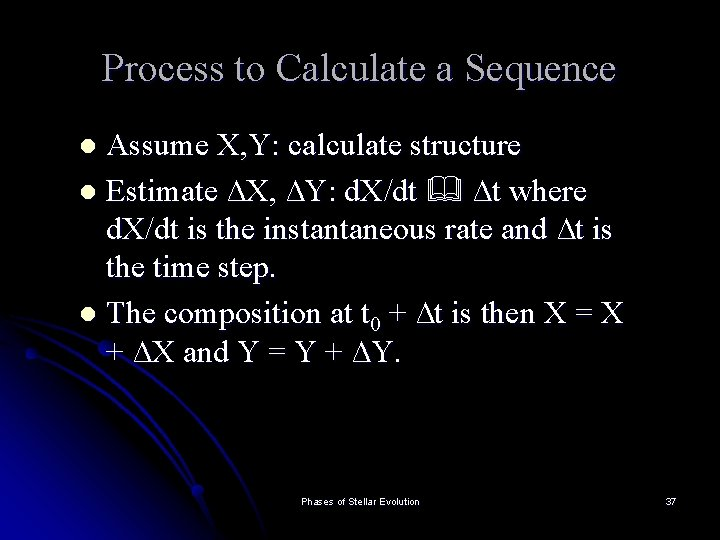Process to Calculate a Sequence Assume X, Y: calculate structure l Estimate ∆X, ∆Y: d. X/dt ∆t where d. X/dt is the instantaneous rate and ∆t is the time step. l The composition at t 0 + ∆t is then X = X + ∆X and Y = Y + ∆Y. l Phases of Stellar Evolution 37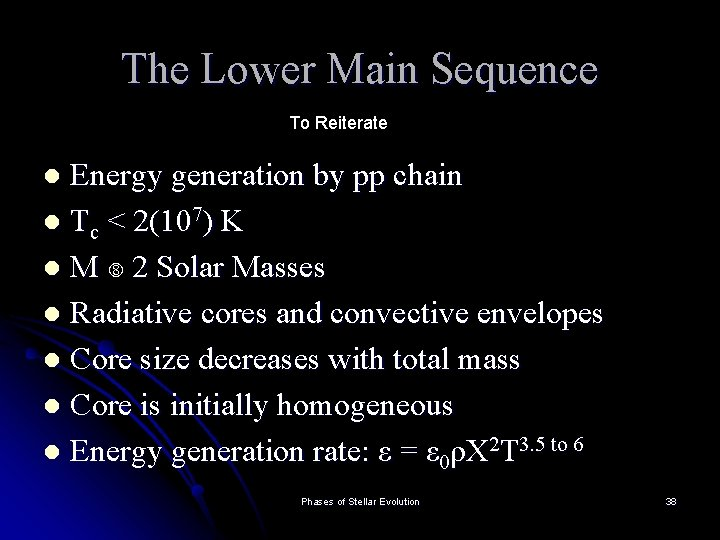The Lower Main Sequence To Reiterate Energy generation by pp chain l Tc < 2(107) K l M 2 Solar Masses l Radiative cores and convective envelopes l Core size decreases with total mass l Core is initially homogeneous l Energy generation rate: ε = ε 0ρX 2 T 3. 5 to 6 l Phases of Stellar Evolution 38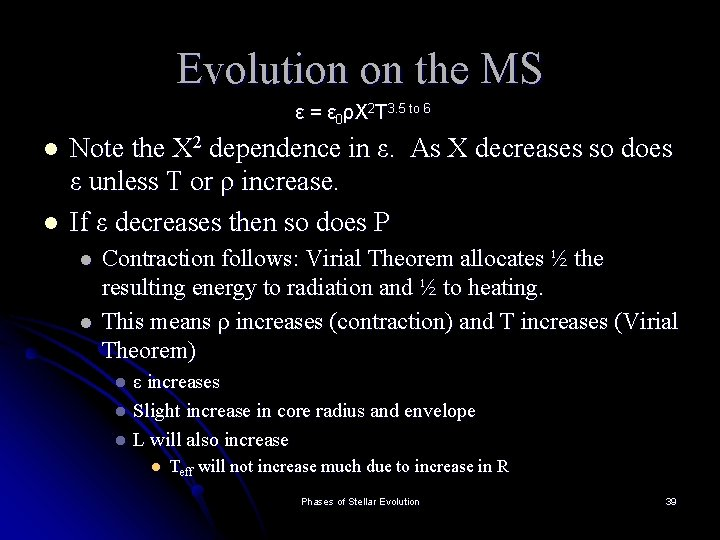Evolution on the MS ε = ε 0ρX 2 T 3. 5 to 6 l l Note the X 2 dependence in ε. As X decreases so does ε unless T or ρ increase. If ε decreases then so does P l l Contraction follows: Virial Theorem allocates ½ the resulting energy to radiation and ½ to heating. This means ρ increases (contraction) and T increases (Virial Theorem) ε increases l Slight increase in core radius and envelope l L will also increase l l Teff will not increase much due to increase in R Phases of Stellar Evolution 39At the Solar Age T = 4. 3 Gigayears l l l Note that 90% of the mass r/R = 0. 5. X normalized to 1: depletion limited to r/R < 0. 3 (0. 5 in m/M) L = L at r = 0. 3 R. Phases of Stellar Evolution 40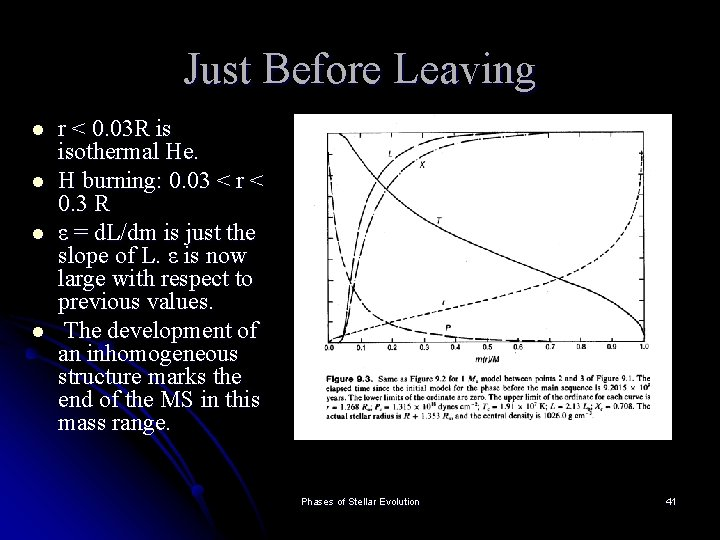Just Before Leaving l l r < 0. 03 R is isothermal He. H burning: 0. 03 < r < 0. 3 R ε = d. L/dm is just the slope of L. ε is now large with respect to previous values. The development of an inhomogeneous structure marks the end of the MS in this mass range. Phases of Stellar Evolution 41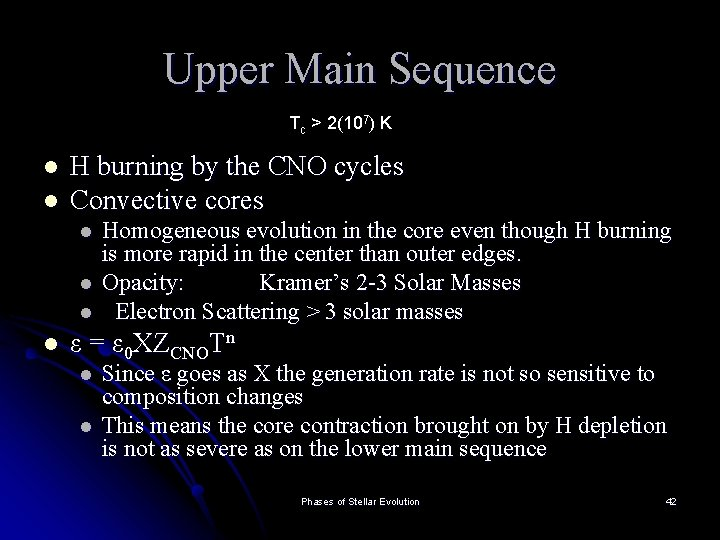Upper Main Sequence Tc > 2(107) K l l H burning by the CNO cycles Convective cores l l Homogeneous evolution in the core even though H burning is more rapid in the center than outer edges. Opacity: Kramer’s 2 -3 Solar Masses Electron Scattering > 3 solar masses ε = ε 0 XZCNOTn l l Since ε goes as X the generation rate is not so sensitive to composition changes This means the core contraction brought on by H depletion is not as severe as on the lower main sequence Phases of Stellar Evolution 42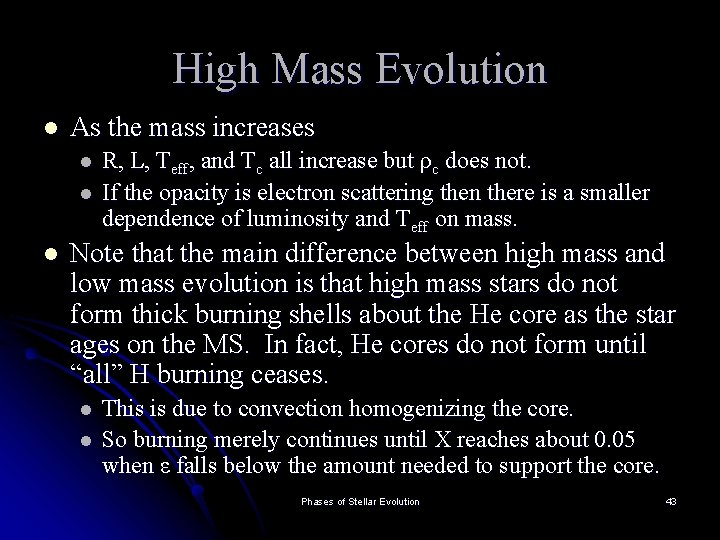High Mass Evolution l As the mass increases l l l R, L, Teff, and Tc all increase but ρc does not. If the opacity is electron scattering then there is a smaller dependence of luminosity and Teff on mass. Note that the main difference between high mass and low mass evolution is that high mass stars do not form thick burning shells about the He core as the star ages on the MS. In fact, He cores do not form until “all” H burning ceases. l l This is due to convection homogenizing the core. So burning merely continues until X reaches about 0. 05 when ε falls below the amount needed to support the core. Phases of Stellar Evolution 43The Isothermal Core l l l Low Mass Stars Only d. L/dr ≈ 4πr 2ρε(r) L(0) = 0 and If ε = 0 throughout a region then L = 0 as well. But d. T/dr ~ L(r) so if L = 0 then T = Constant So what supports the core (it is NOT degenerate)? A steep density gradient. There is a limiting mass for this case - it is called the Schönberg-Chandrasekhar limit and is approximately 0. 12 solar masses. l l l A core with Mc < MSC is stable but a burning shell above it will continually add mass. The result is that the limit will be exceeded. The core will start to collapse Phases of Stellar Evolution 44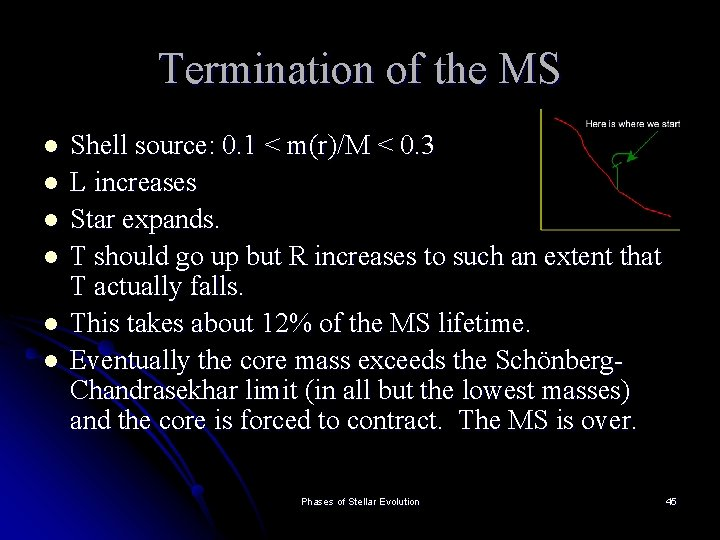Termination of the MS l l l Shell source: 0. 1 < m(r)/M < 0. 3 L increases Star expands. T should go up but R increases to such an extent that T actually falls. This takes about 12% of the MS lifetime. Eventually the core mass exceeds the Schönberg. Chandrasekhar limit (in all but the lowest masses) and the core is forced to contract. The MS is over. Phases of Stellar Evolution 45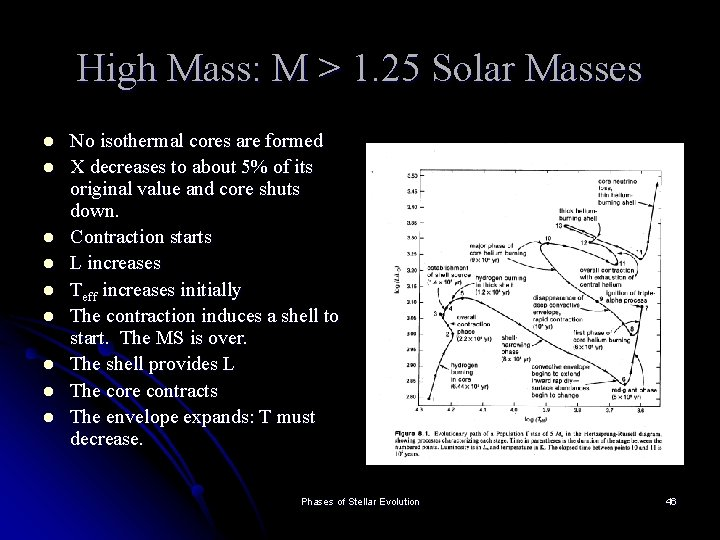High Mass: M > 1. 25 Solar Masses l l l l l No isothermal cores are formed X decreases to about 5% of its original value and core shuts down. Contraction starts L increases Teff increases initially The contraction induces a shell to start. The MS is over. The shell provides L The core contracts The envelope expands: T must decrease. Phases of Stellar Evolution 46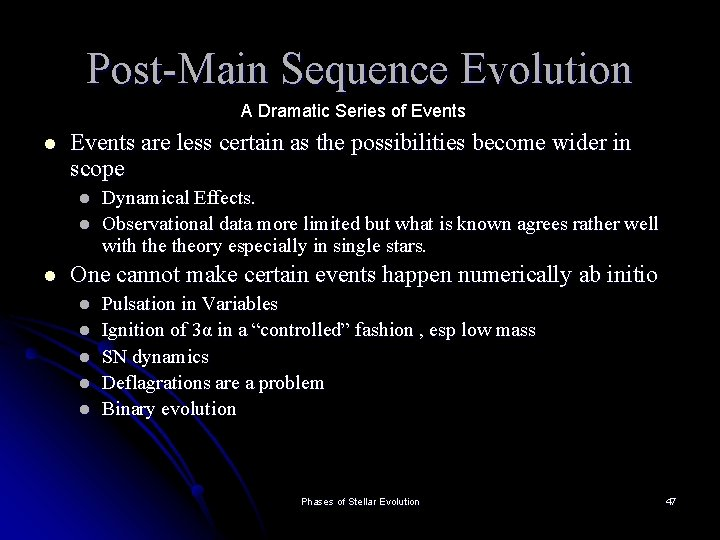Post-Main Sequence Evolution A Dramatic Series of Events l Events are less certain as the possibilities become wider in scope l l l Dynamical Effects. Observational data more limited but what is known agrees rather well with theory especially in single stars. One cannot make certain events happen numerically ab initio l l l Pulsation in Variables Ignition of 3α in a “controlled” fashion , esp low mass SN dynamics Deflagrations are a problem Binary evolution Phases of Stellar Evolution 47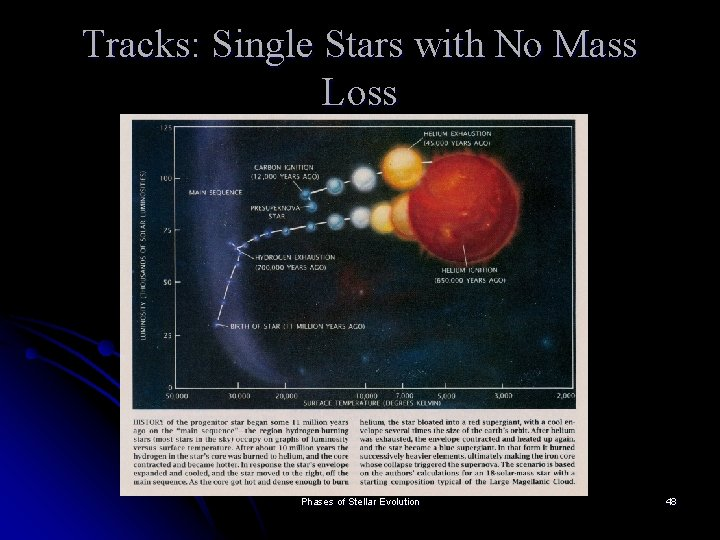Tracks: Single Stars with No Mass Loss Phases of Stellar Evolution 48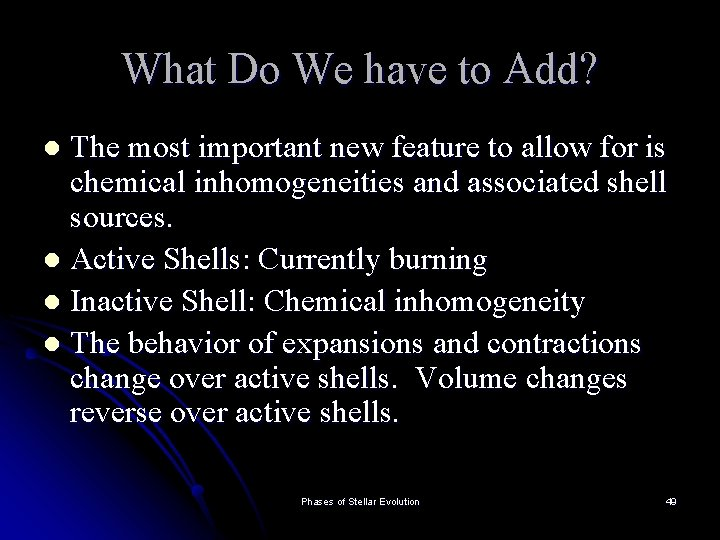What Do We have to Add? The most important new feature to allow for is chemical inhomogeneities and associated shell sources. l Active Shells: Currently burning l Inactive Shell: Chemical inhomogeneity l The behavior of expansions and contractions change over active shells. Volume changes reverse over active shells. l Phases of Stellar Evolution 49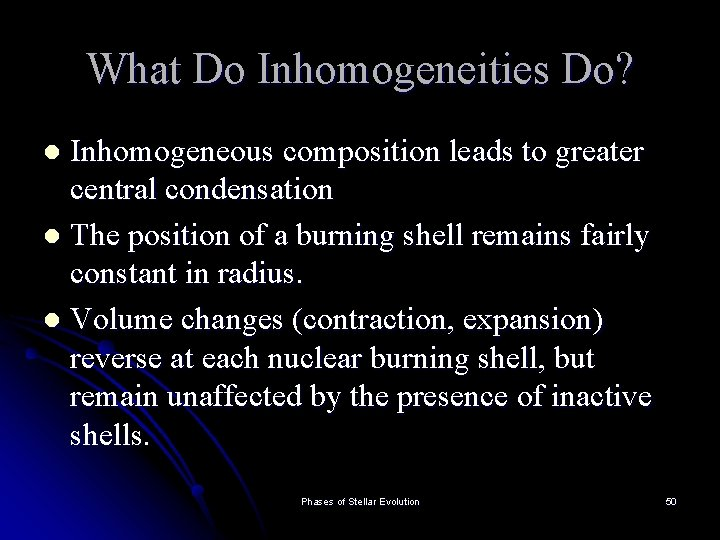What Do Inhomogeneities Do? Inhomogeneous composition leads to greater central condensation l The position of a burning shell remains fairly constant in radius. l Volume changes (contraction, expansion) reverse at each nuclear burning shell, but remain unaffected by the presence of inactive shells. l Phases of Stellar Evolution 50Desidera l l Nuclear ash has (usually) μ > μfuel Larger μ ==> larger central core values for ρ Note that the core γ = 4/3 (n = 3). Stationary shells: l l If it tries to burn inwards then T increases and so does ε which means that P will increase forcing the shell back out. Also trying to burn inwards means that one is moving to zones depleted in the current fuel. Cannot go out (in radius) because T will be too low to support the burning. Phases of Stellar Evolution 51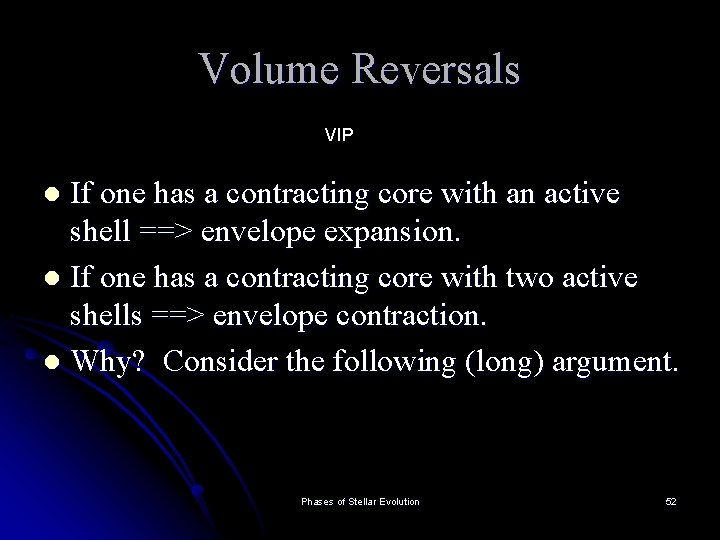Volume Reversals VIP If one has a contracting core with an active shell ==> envelope expansion. l If one has a contracting core with two active shells ==> envelope contraction. l Why? Consider the following (long) argument. l Phases of Stellar Evolution 52Central Condensation l l A measure of the central condensation for a particular volume is the ratio ρ(r) (the local value of the density) to <ρ(r)> the mean density of the material interior to r. We define U ≡ 3ρ(r)/<ρ(r)> where <ρ(r)> = 3 m(r) / 4πr 3 One can show: U = d(ln(m(r))/d(ln(r)) = d ln(q) / d ln(r) where q ≡ m(r)/M What are the limits on U? l l At r = R ρ(r) = 0 U = 0 at the upper boundary At r = 0 ρ(r) = ρc and <ρ(r)> = ρc U = 3 Phases of Stellar Evolution 53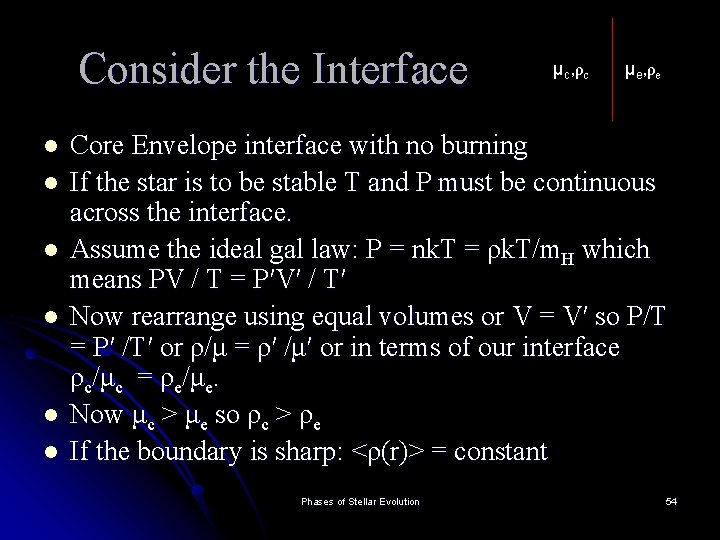Consider the Interface l l l μc, ρc μe, ρe Core Envelope interface with no burning If the star is to be stable T and P must be continuous across the interface. Assume the ideal gal law: P = nk. T = ρk. T/m. H which means PV / T = P′V′ / T′ Now rearrange using equal volumes or V = V′ so P/T = P′ /T′ or ρ/μ = ρ′ /μ′ or in terms of our interface ρc / μ c = ρe / μ e. Now μc > μe so ρc > ρe If the boundary is sharp: <ρ(r)> = constant Phases of Stellar Evolution 54Interfaces l l l Note that if there is a ρ(r) discontinuity then there is a U(r) discontinuity Since U ≡ 3ρ(r)/<ρ(r)> and <ρ(r)> = constant for a sharp interface U goes as ρ. This means since ρc/μc = ρe/μe that Uc/μc = Ue/μe. There are two additional characterizations: l l V ≡ -d ln. P/ d lnr (Pressure scale height) N+1 ≡ d ln. P/ d lnr = -V These can be evaluated adiabatically and if done that way N is related to 2 An adiabatic process is one in there is no heat flow l A free expansion is an adiabatic process l l No Heat Flow No Work Phases of Stellar Evolution 55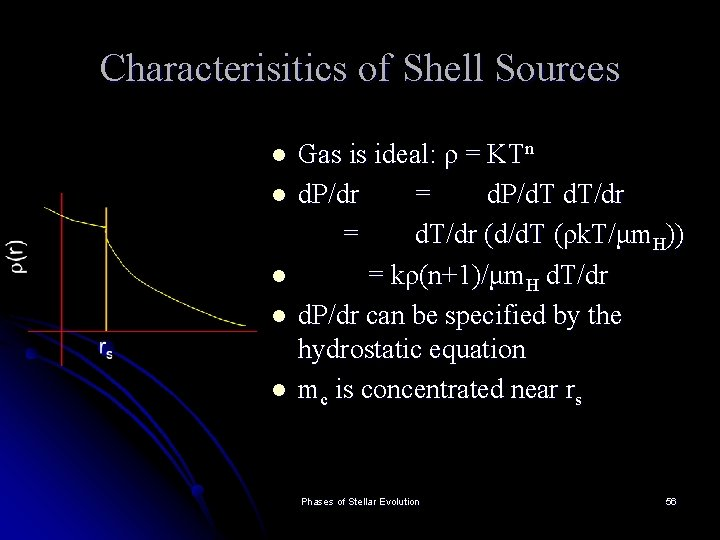Characterisitics of Shell Sources l l l Gas is ideal: ρ = KTn d. P/dr = d. P/d. T/dr = d. T/dr (d/d. T (ρk. T/μm. H)) = kρ(n+1)/μm. H d. T/dr d. P/dr can be specified by the hydrostatic equation mc is concentrated near rs Phases of Stellar Evolution 56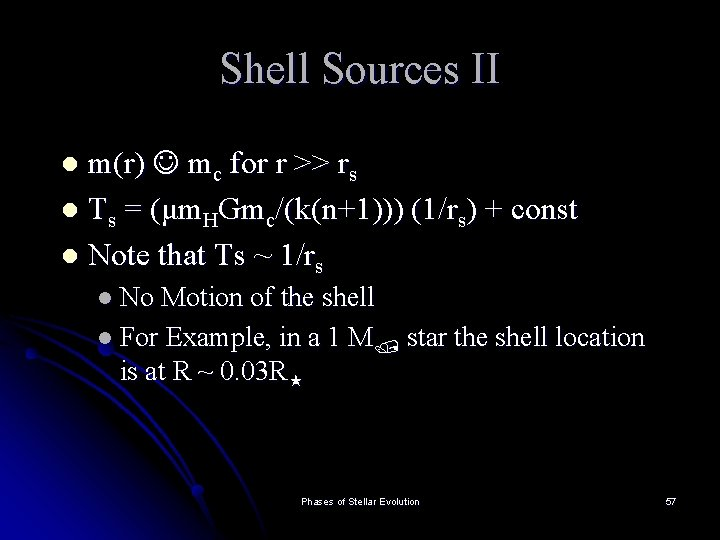Shell Sources II m(r) mc for r >> rs l Ts = (μm. HGmc/(k(n+1))) (1/rs) + const l Note that Ts ~ 1/rs l l No Motion of the shell l For Example, in a 1 M star the shell location is at R ~ 0. 03 R Phases of Stellar Evolution 57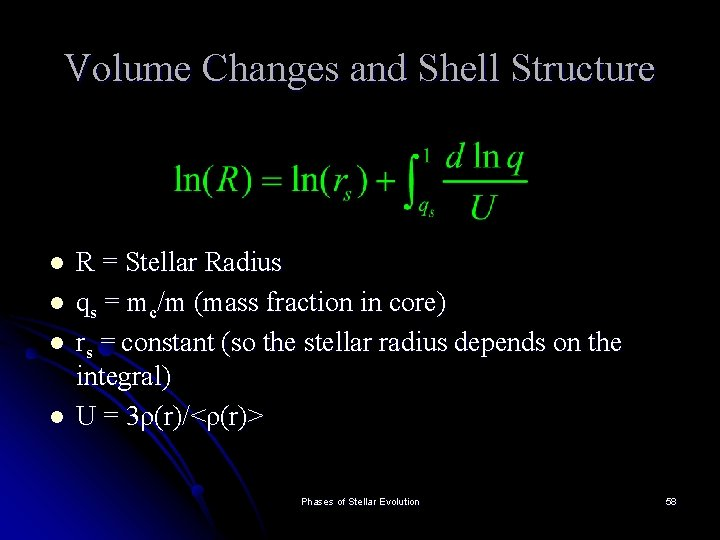Volume Changes and Shell Structure l l R = Stellar Radius qs = mc/m (mass fraction in core) rs = constant (so the stellar radius depends on the integral) U = 3ρ(r)/<ρ(r)> Phases of Stellar Evolution 58What Happens? l l l Consider ρc increasing due to core condensation rs remains fixed. ρ at the edge of the shell (inside) will decrease <ρ(rs)> is constant (to first order : ∆M = 0) Therefore we have a decrease in Uc but if Uc decreases so must Ue Therefore: reduce U(r) in the lowest levels of the envelope where 1/q is largest ==> decrease the density. Phases of Stellar Evolution 59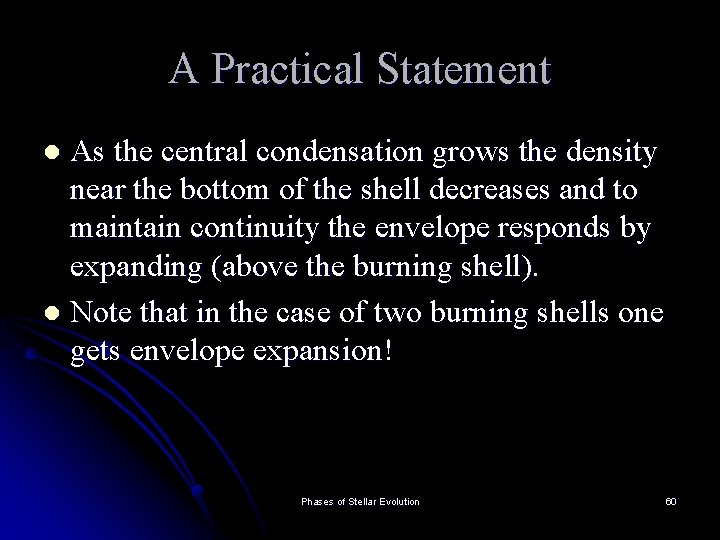A Practical Statement As the central condensation grows the density near the bottom of the shell decreases and to maintain continuity the envelope responds by expanding (above the burning shell). l Note that in the case of two burning shells one gets envelope expansion! l Phases of Stellar Evolution 60